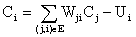# 洛谷1038 神经网络

## 比赛前刷水题

Posted by yjjr's blog on March 8, 2019

# 题目

## 题目描述$C_i=\sum_{j,i \in E} W_{ji}C_{j}-U{i}$

## 输入输出样例

### 输入样例#1

5 6
1 0
1 0
0 1
0 1
0 1
1 3 1
1 4 1
1 5 1
2 3 1
2 4 1
2 5 1


### 输出样例#1

3 1
4 1
5 1


SOI 2T前来刷水了！！！

## Code

#include<iostream>
#include<cstdio>
#include<cstdlib>
#include<cmath>
#include<cstring>
#include<algorithm>
#define rep(i,a,b) for(int i=a;i<=b;i++)
#define dep(i,a,b) for(int i=a;i>=b;i--)
#define ll long long
#define mem(x,num) memset(x,num,sizeof x)
#define reg(x) for(int i=last[x];i;i=e[i].next)
using namespace std;
inline ll read(){
ll f=1,x=0;char ch=getchar();
while(ch<'0'||ch>'9'){if(ch=='-')f=-1;ch=getchar();}
while(ch>='0'&&ch<='9'){x=x*10+ch-'0';ch=getchar();}
return x*f;
}
//******head by yjjr******
const int maxn=1e4+6;
int n,m,cnt=0,c[maxn],last[maxn],u[maxn],du[maxn],chu[maxn],que[maxn<<2];
bool vis[maxn];
struct edge{int to,next,w;}e[maxn<<1];
void insert(int x,int y,int w){e[++cnt]=(edge){y,last[x],w};last[x]=cnt;}
void topsort(){
int head=0,tail=0;
rep(i,1,n)if(!du[i])que[++tail]=i;
while(head<=tail){
int now=que[head++];
reg(now){
du[e[i].to]--;
if(c[now]>0)c[e[i].to]+=c[now]*e[i].w;
if(!du[e[i].to])que[++tail]=e[i].to;
}
}
}
int main(){
n=read(),m=read();
rep(i,1,n){
c[i]=read(),u[i]=read();
if(c[i]==0)c[i]=-u[i];
}
rep(i,1,m){
int x=read(),y=read(),z=read();
insert(x,y,z);du[y]++;vis[x]=1;
}
//rep(i,1,n)cout<<c[i]<<' '<<u[i]<<endl;
topsort();
bool flag=0;
rep(i,1,n)if(c[i]>0&&vis[i]==0)printf("%d %d\n",i,c[i]),flag=1;
if(!flag)puts("NULL");
return 0;
}